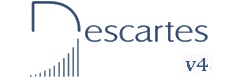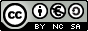Multiples and factors. Prime numbers First and Second Year of Secondary Education

The  factors of a number.

The factors of a natural number are the natural numbers that divide exactly into it, resulting in another natural number in the quotient and 0 in the remainder.

Esta unidad interactiva requiere la máquina virtual de Java J2RE.
 Type any number in the control box below and hit Enter. In this way you will get all the factors of that number. You can also change the value of the number by using the up and down triangles. The largest number you can type is 10.000.

Investigate.

1.- Write down the quantity of factors of each number from 0 to 50.
What is the natural number that has the highest number of factors? How many factors does it have?
What is the natural number that has the lowest number of factors? How many factors does it have?
How many factors does a prime number have?

2.-  Define four sections to make the classification of natural numbers; depending on the number of factors, write a number as an example of each of those sections.

3.- Type a number of 4 digits and hit Enter; use the up triangle to increase the value of the number by one at the time. Notice the diversity of the number of factors of each number. Write down what you observe. Do you think that a large number is expected to have more factors? What do you think it depends on?

 Eduardo Barbero CorralSpanish Ministry of Education. Year 2007